Question

# Calculate molality of a 10.0% NaCl (aq) (MM= 58.44g/mol) please write out steps so I can...

Calculate molality of a 10.0% NaCl (aq) (MM= 58.44g/mol)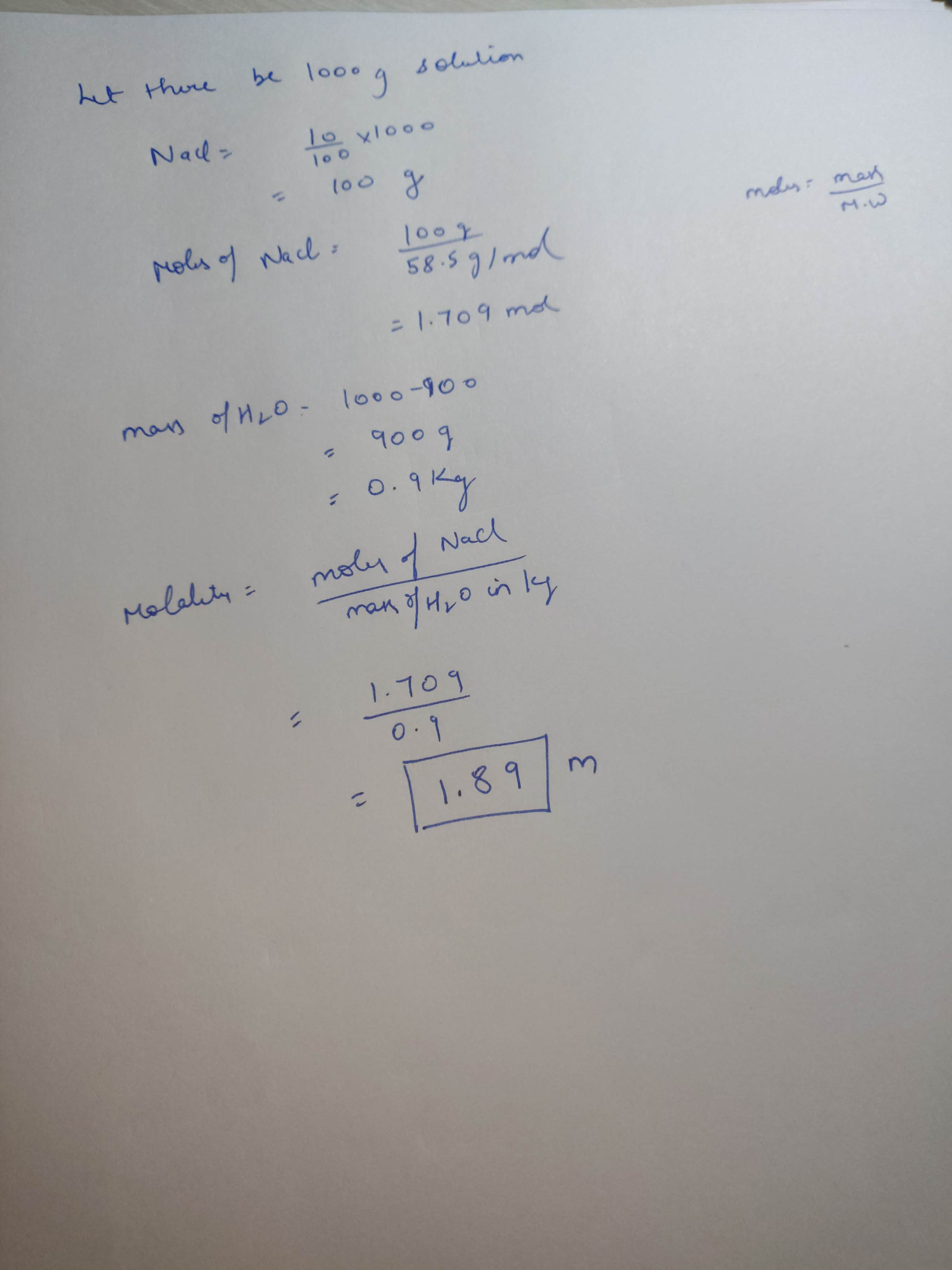#### Earn Coins

Coins can be redeemed for fabulous gifts.

Similar Homework Help Questions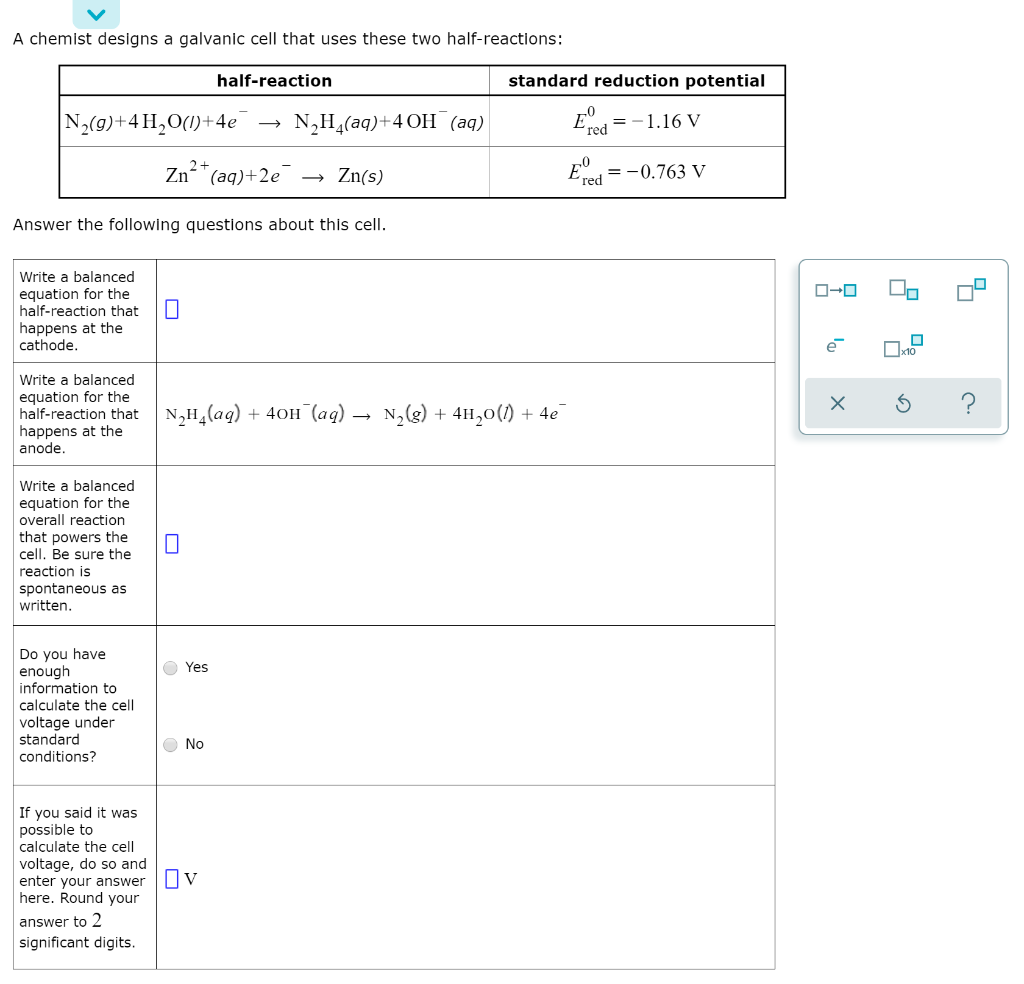Can someone please help me out with this problem? If possible could you add steps so that I can be able to do the rest on my own? Thank YOUUUU!! A chemist designs a galvanic cell that uses these two half-reactions: half-reaction standard reduction potential N (9)+4H2O(1)+4e → N H (aq)+4 OH (aq) Ered = -1.16 V Zn²+ (aq)+2e → Zn(s) Exped=-0.763 V Answer the following questions about this cell. Write a balanced equation for the half-reaction that happens at...

please help, with CLEAR steps so I can follow along please! 1a. 5.0 g of O2 (g) and 5.0 g of N2 (g), both at 35°C, are added to a 350-mL container. Calculate the total pressure in the container. Express answer in atm. b. When 0.500 g zinc is added to excess hydrochloric acid, H2 gas is formed. Calculate the volume of gas formed at STP, assuming 100% yield. Zn (s) + 2HCl (aq) —> ZnCl2 (aq) + H2 (g)

• ### Computer Systems/Architecture: Please explain your steps and write clearly so I can follow along and learn....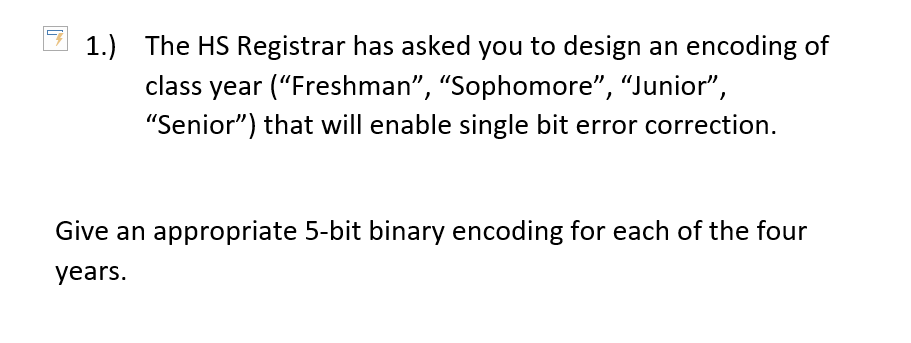Computer Systems/Architecture: Please explain your steps and write clearly so I can follow along and learn. I will rate right after, thanks! 1.) The HS Registrar has asked you to design an encoding of class year ("Freshman", "Sophomore", "Junior", "Senior") that will enable single bit error correction. Give an appropriate 5-bit binary encoding for each of the four years.

• ### Please list out all the steps and equations used so I understand. Also, if you do...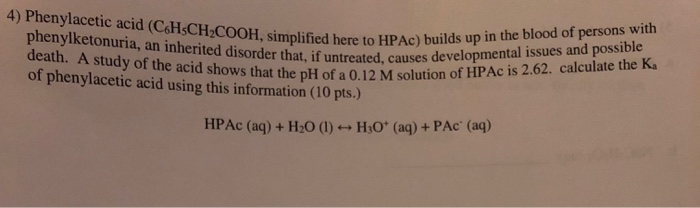Please list out all the steps and equations used so I understand. Also, if you do not have legible handwriting then please don't write it out and upload.​ 4) Phenylacetic acid (CaHsCH COOH, simplified here to HPAc) builds up n COOH, simplified here to HIP phenylketonuria, an inherited disorder that, if untreated, causes of phenylacetic acid using this information (10 pts.) at the pH of a 0.12 M solution of HPAc is 2.62. calculate the Ka НРАс (aq) + H2O...

• ### please help thanks Calculate Ksp for the salt NaCl at 25°C. Substance Na+(aq) Cl'(aq) NaCl(s) DGfº(in kJ/mol) -2620...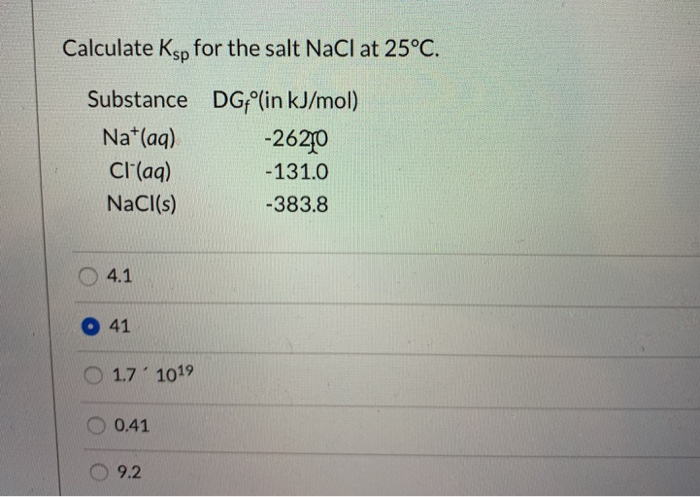please help thanks Calculate Ksp for the salt NaCl at 25°C. Substance Na+(aq) Cl'(aq) NaCl(s) DGfº(in kJ/mol) -2620 -131.0 -383.8 4.1 O 41 O 1.7' 1019 O 0.41 O 9.2

• ### Please write clearly so I am able to follow the steps and understand how this problem...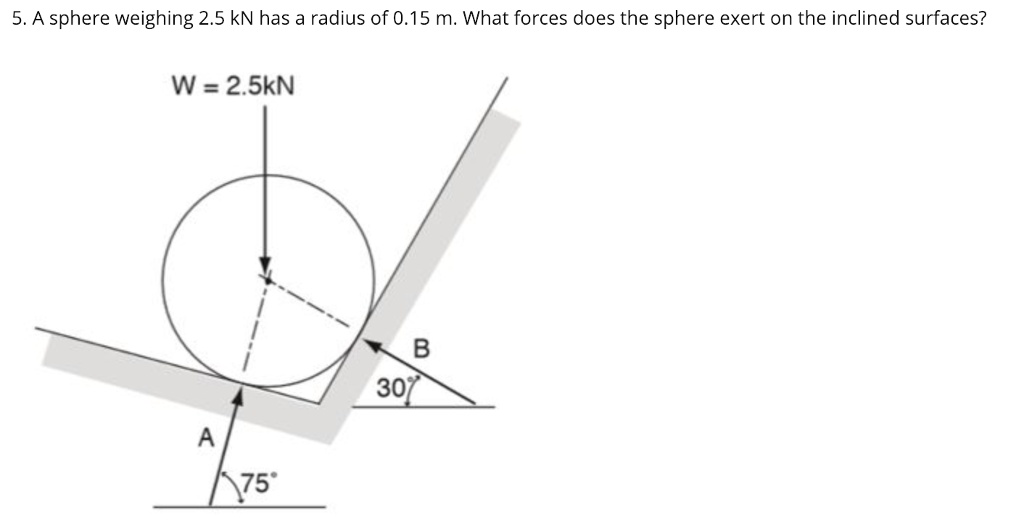Please write clearly so I am able to follow the steps and understand how this problem is done. Thank you! 5. A sphere weighing 2.5 kN has a radius of 0.15 m. What forces does the sphere exert on the inclined surfaces? W-2.5kN 30 75

• ### please show steps so i can follow along -Solve the equation 8*+3 = 42x-1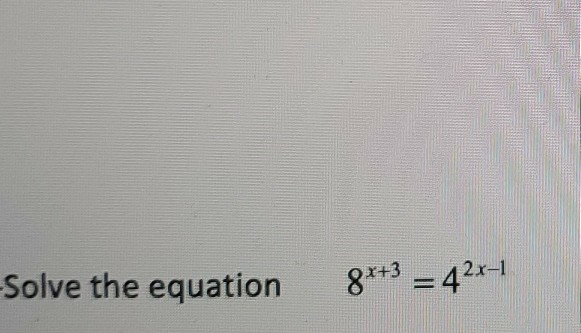please show steps so i can follow along -Solve the equation 8*+3 = 42x-1

• ### please write step by step so i can follow along. thank you 4) Let (2) =...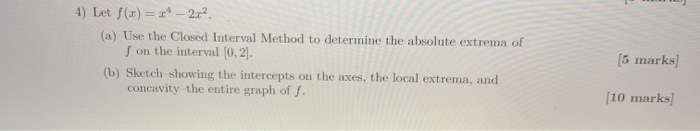please write step by step so i can follow along. thank you 4) Let (2) = 2* - 202. (a) Use the Closed Interval Method to determine the absolute extrema of S on the interval (0,2). (b) Sketch showing the intercepts on the axes, the local extrema, and concavity the entire graph of f. (5 marks) [10 marks]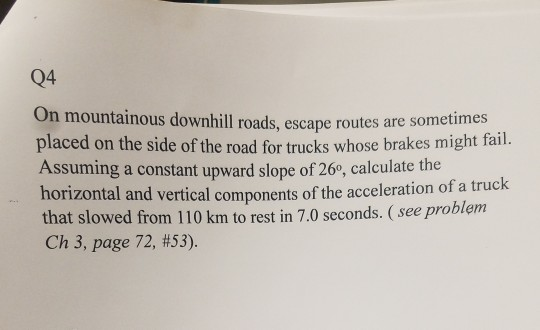please help me answer this question and also please write clearly so I can understand thank you so much also please show all steps thank you so much Q4 On mountainous downhill roads, escape routes are sometimes placed on the side of the road for t Assuming a constant upward slope of 26, calculate the horizontal that slowed from 110 km to rest in 7.0 seconds. (see proble Ch 3, page 72, #53). rucks whose brakes might fail. and vertical...

• ### Q) Hi, Can you please answer the question using clear detailed steps and definitions so I...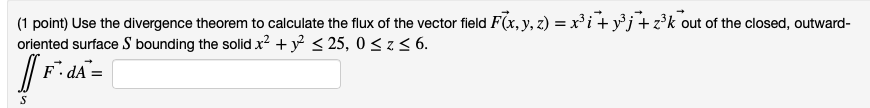Q) Hi, Can you please answer the question using clear detailed steps and definitions so I can better understand it? Thank you so much! :) (1 point) Use the divergence theorem to calculate the flux of the vector field F(x, y, z) = xi + y’j + zk out of the closed, outward- oriented surface S bounding the solid x2 + y2 < 25, 0 <236. FdA=